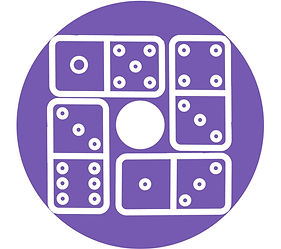# Use your calculation skills to solve these domino donut puzzles.

### GOAL

The goal of domino donuts is to place the four given domino pieces so the number of spots (also called pips, nips, or dobs) on the dominos are all equal on each side of the square.

### stEPS

1. Position the dominoes shown for each puzzle so that the sum of all the dots on each side equals 14.

2. If there's a question mark on a domino, figure out what the domino should be.

Ready for a challenge? Try this domino game online.

PUZZLE SOLUTIONS